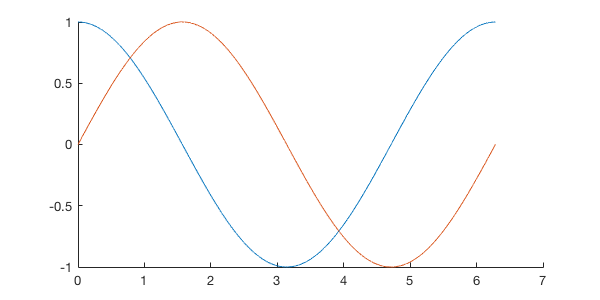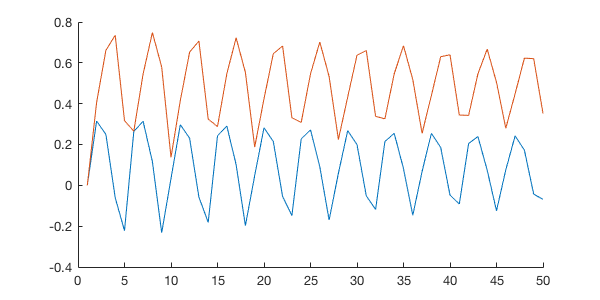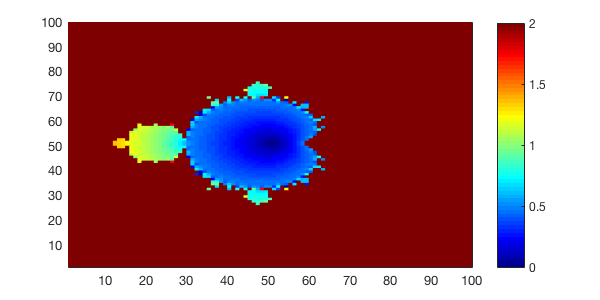# Lecture 5: Random Numbers & Complex Numbers¶

Date: 09/14/2017, Thursday

In :

format compact


## Built-in random number generator¶

rand returns a random number between [0,1] (uniform distribution).

In :

rand

ans =
0.8147


rand(N) returns a $$N \times N$$ random matrix. You can always type doc rand or help rand to see the detailed usage.

In :

rand(5)

ans =
0.9058    0.2785    0.9706    0.4218    0.0357
0.1270    0.5469    0.9572    0.9157    0.8491
0.9134    0.9575    0.4854    0.7922    0.9340
0.6324    0.9649    0.8003    0.9595    0.6787
0.0975    0.1576    0.1419    0.6557    0.7577


randi(N) returns an integer between 1 and N.

In :

randi(100)

ans =
75


randn uses normal distribution, instead of uniform distribution.

In :

randn(5)

ans =
-0.3034   -1.0689   -0.7549    0.3192    0.6277
0.2939   -0.8095    1.3703    0.3129    1.0933
-0.7873   -2.9443   -1.7115   -0.8649    1.1093
0.8884    1.4384   -0.1022   -0.0301   -0.8637
-1.1471    0.3252   -0.2414   -0.1649    0.0774


## Complex numbers¶

### Complex number basics¶

A real number (double-precision) takes 8 Bytes (64 bits). A complex number is a pair of numbers so simply takes 16 Bytes (128 bits)

In :

x = 3;
y = 4;
z = x+i*y;

In :

whos

  Name      Size            Bytes  Class     Attributes

ans       5x5               200  double
x         1x1                 8  double
y         1x1                 8  double
z         1x1                16  double    complex



Take conjugate of a complex number:

In :

conj(z)

ans =
3.0000 - 4.0000i


Show the number on the complex plane

In :

%plot --size 300,300
hold on
complex_number_plot_fn(conj(z)/5,'r') % complex_number_plot_fn is available on canvas.
complex_number_plot_fn(z/5,'b')3 equivalent ways to compute $$|z|$$

In :

abs(z)

ans =
5

In :

sqrt(dot(z,z))

ans =
5

In :

norm(z)

ans =
5


The angle of z in degree:

In :

angle(z) / pi * 180.0

ans =
53.1301


### Euler’s Formula¶

$e^{i\theta} = \cos(\theta) + i\sin(\theta)$

Verify that MATLAB understands $$e^{i\theta}$$

In :

theta = linspace(0, 2*pi, 1e4);
z = exp(i*theta); % now z is an array, not a scalar as defined in the previou section.

In :

%plot --size 600,300
hold on
plot(theta,real(z))
plot(theta,imag(z))### Mandelbrot set¶

#### Iteration with a single parameter¶

In :

c = rand-0.5 + i*(rand-0.5);

T = 50;
z_arr = zeros(T,1); % to hold the entire time series

z = 0; % initial value
z_arr(1) = z;

for t=2:T
z = z^2+c;
z_arr(t) = z;
end

In :

hold on
plot(real(z_arr))
plot(imag(z_arr))Run this code repeatedly, you will see sometimes $$z$$ will blow up, sometime not, according to the initial value of $$c$$. Thus we want to figure out what values of $$c$$ will make $$z$$ blow up.

#### Iteration with the entire paremeter space¶

We want to construct a 2D array containing all possible values of $$c$$ on the complex plane.

Let’s make 1D grids first.

In :

nx = 100;
xm = 1.75;
x = linspace(-xm, xm, nx);
y = linspace(-xm, xm, nx);

In :

size(x), size(y)

ans =
1   100
ans =
1   100


convert 1D grid to 2D grid.

In :

[Cr, Ci] = meshgrid(x,y);

In :

size(Cr), size(Ci)

ans =
100   100
ans =
100   100

In :

C = Cr + i*Ci; % now C spans over the complex plane

In :

size(C)

ans =
100   100


Run the iteration for every value of C

In :

T = 50;

Z_final = zeros(nx,nx); % to hold last value of z, at all possible points.

for ix = 1:nx
for iy = 1:nx % we also have nx points in the y-direction

% get the value of c at current point.
% note that MATLAB is case-sensitive
c = C(ix,iy);

z = 0; % initial value, doesn't matter too much
for t=2:T
z = z^2+c;
end
Z_final(ix,iy) = z; % save the last value of z

end
end


Here’s one way to visualize the result.

In :

pcolor(abs(Z_final)); % plot the magnitude of z